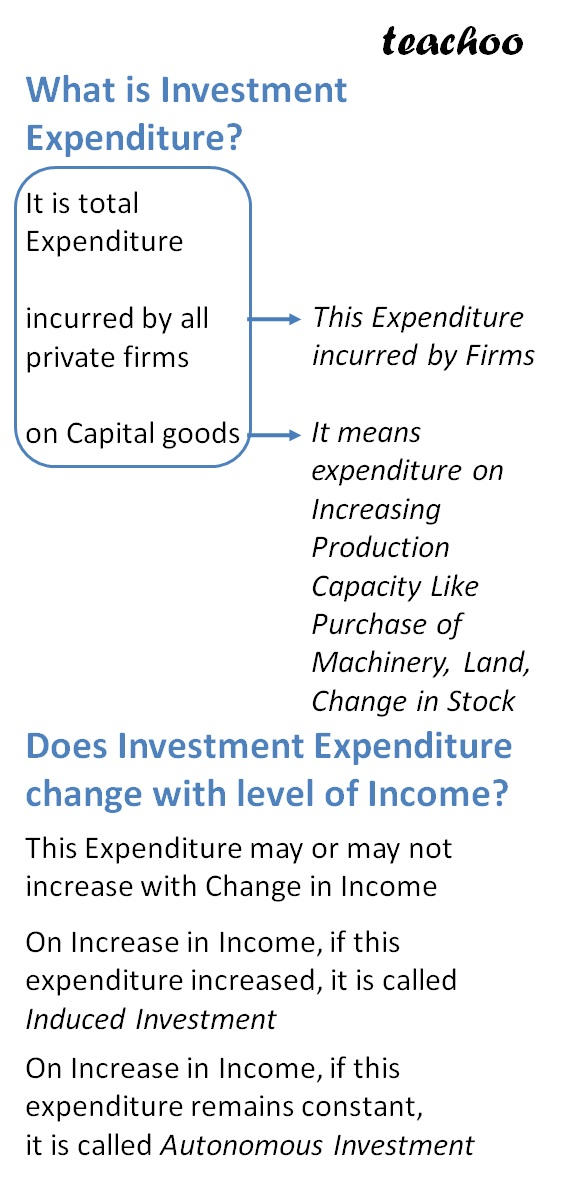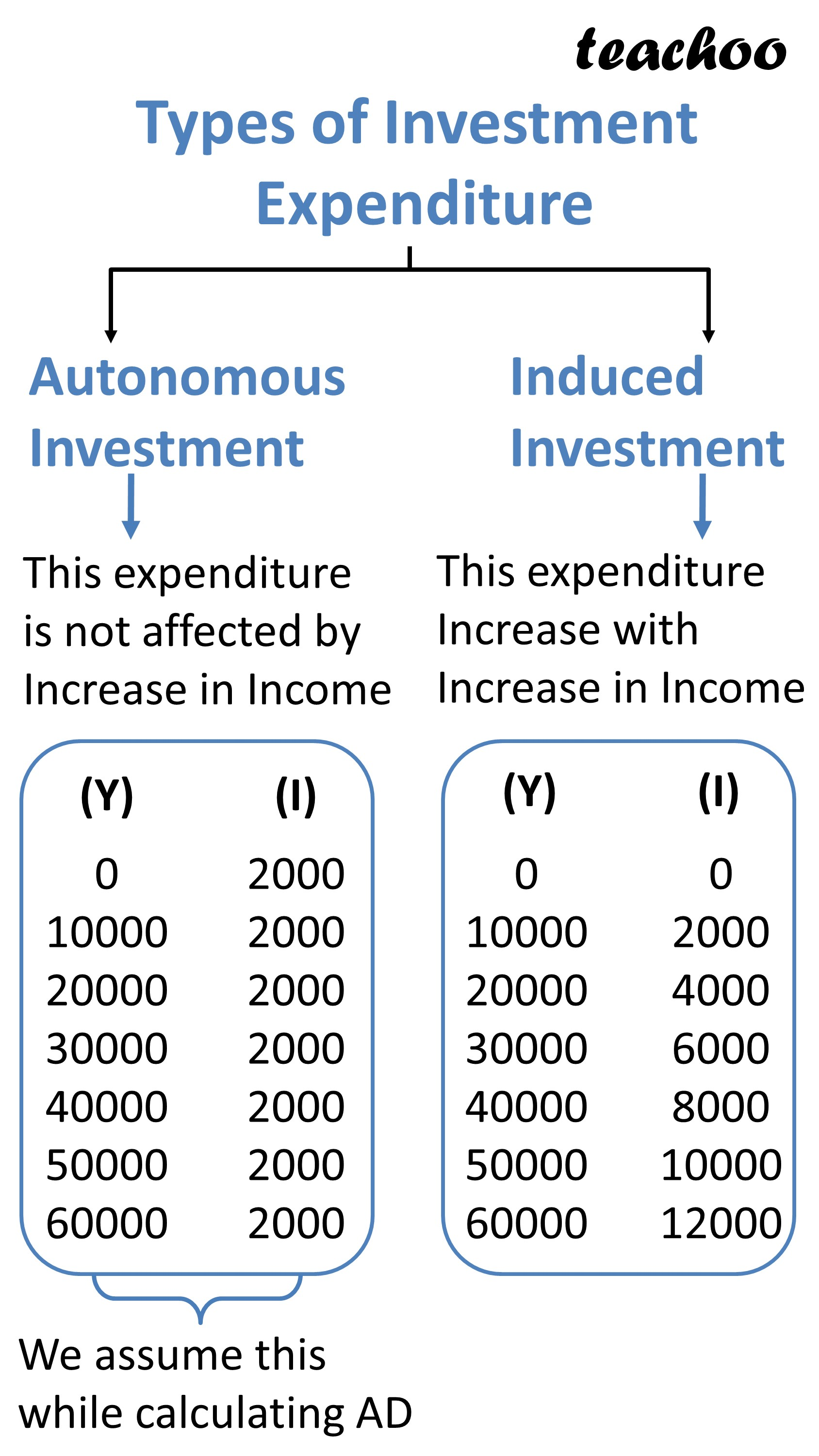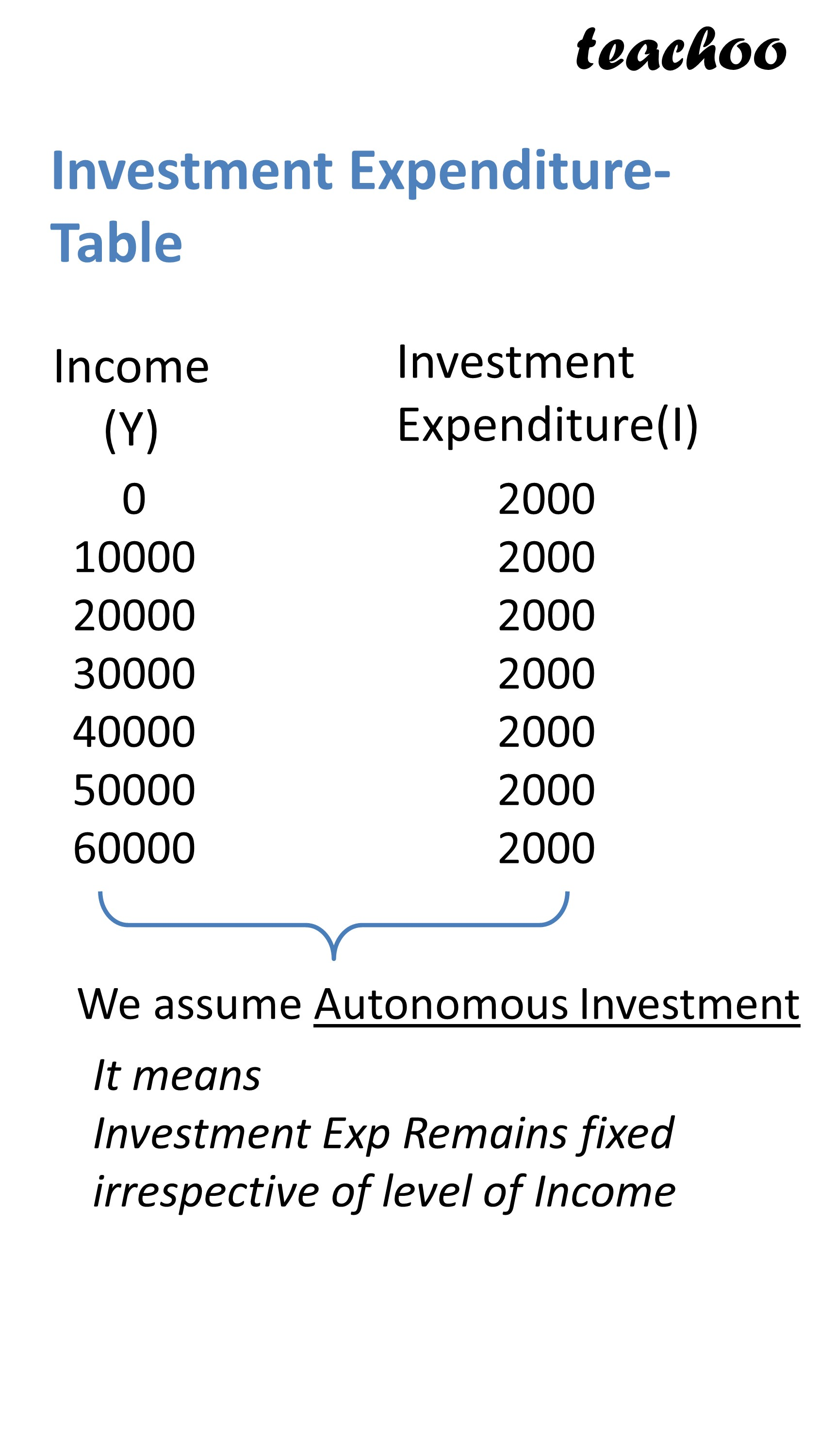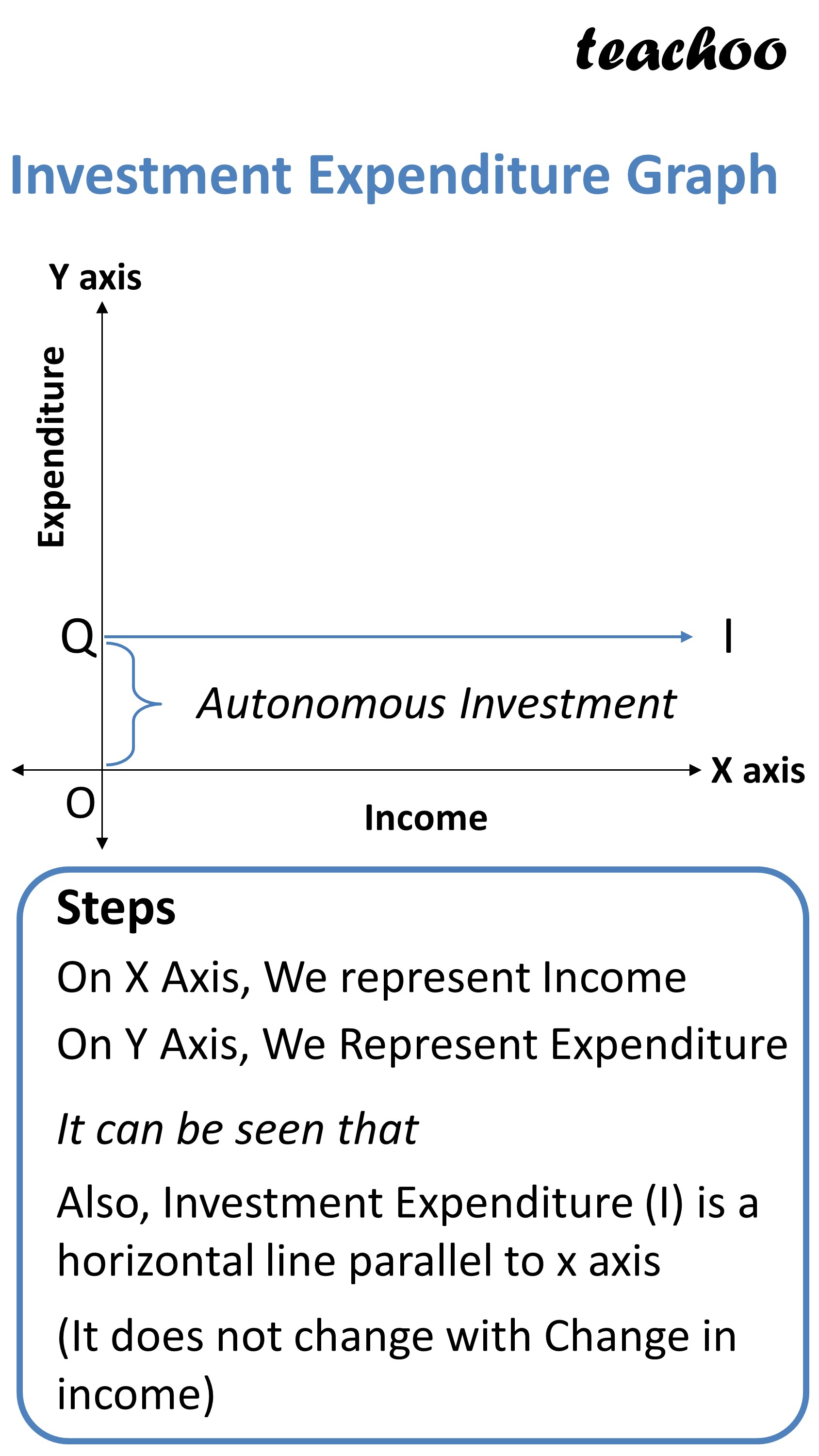Chapter 4 Part 1 - AD,AS and Related Concepts

Economics Class 12
Macroeconomics

## Investment Expenditure

It refers to total expenditure incurred by all firms on Capital Goods

It includes expenditures such as Investment in land, Plant and Machinery and Change in Inventory (Closing Stock - Opening Stock)## Does Investment Expenditure change with level of income?

For the purpose of this chapter,

We will assume that Investment Expenditure do not change with level of income

(Investment Expenditure is Autonomous)

Also, even at 0 level of income, there will be some investment expenditure

Table and Graph of Investment Expenditure### NCERT Questions

No questions in this part

### Other Books

#### Question 1

What is Investment expenditure?

Does Investment Expenditure change with level of income?

Learn in your speed, with individual attention - Teachoo Maths 1-on-1 Class

### Transcript

What is Investment Expenditure? It is total Expenditure incurred by all private firms on Capital goods This Expenditure incurred by Firms It means expenditure on Increasing Production Capacity Like Purchase of Machinery, Land, Change in Stock Does Investment Expenditure change with level of Income? This Expenditure may or may not increase with Change in Income On Increase in Income, if this expenditure increased, it is called Induced Investment On Increase in Income, if this expenditure remains constant, it is called Autonomous Investment Types of Investment Expenditure Autonomous Investment Induced Investment This expenditure is not affected by Increase in Income This expenditure Increase with Increase in Income 0 10000 20000 30000 40000 50000 60000 2000 2000 2000 2000 2000 2000 2000 0 10000 20000 30000 40000 50000 60000 0 2000 4000 6000 8000 10000 12000 We assume this while calculating AD Investment Expenditure- Table Income (Y) 0 10000 20000 30000 40000 50000 60000 Investment Expenditure(I) 2000 2000 2000 2000 2000 2000 2000 We assume Autonomous Investment It means Investment Exp Remains fixed irrespective of level of Income Investment Expenditure Graph Expenditure Autonomous Investment Income X axis Autonomous Investment Steps On X Axis, We represent Income On Y Axis, We Represent Expenditure It can be seen that Also, Investment Expenditure (I) is a horizontal line parallel to x axis (It does not change with Change in income)